GCSE Maths Algebra

Interpreting Graphs

# Interpreting Graphs

Here we will learn about interpreting graphs, including how to plot graphs, how to recognise different graphs, the key features of a graph, as well as sketching and transforming graphs.

There are also interpreting graphs worksheets based on Edexcel, AQA and OCR exam questions, along with further guidance on where to go next if you’re still stuck.

## What is interpreting graphs?

Interpreting graphs covers the skills of plotting and sketching a variety of different graphs as well as identifying the shapes and key features of those graphs. It also covers transformations of graphs.

In GCSE maths, we study the following types of graphs,

• Linear graphs
• Cubic graphs
• Reciprocal graphs
• Exponential graphs (higher only)
• Trigonometric graphs (higher only)
• Graphs of circles (higher only)

Each type of graph has a specific shape and features which we need to be familiar with.

### What is interpreting graphs?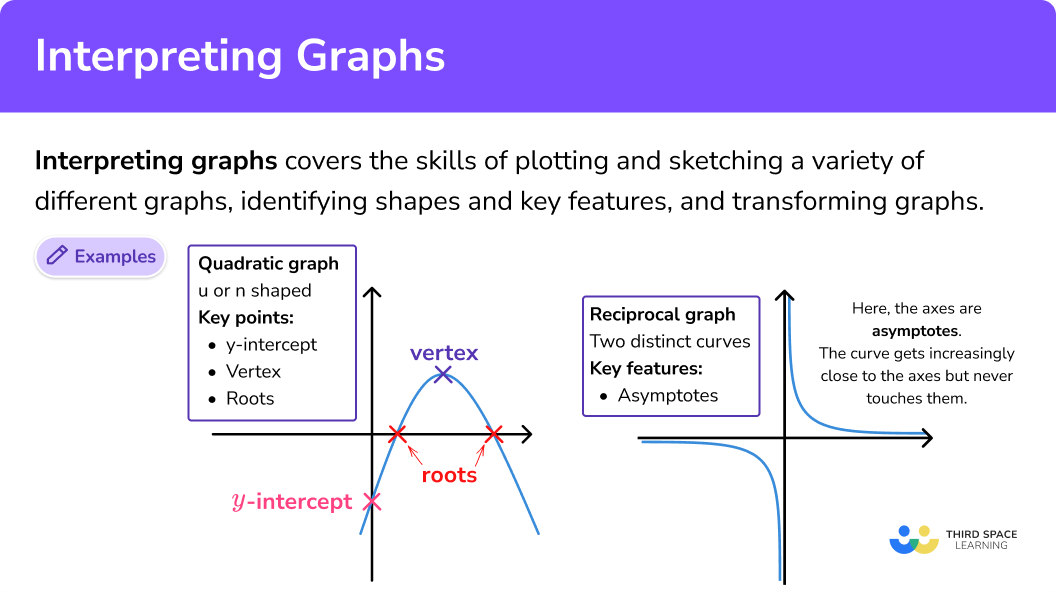### What are the different types of graphs?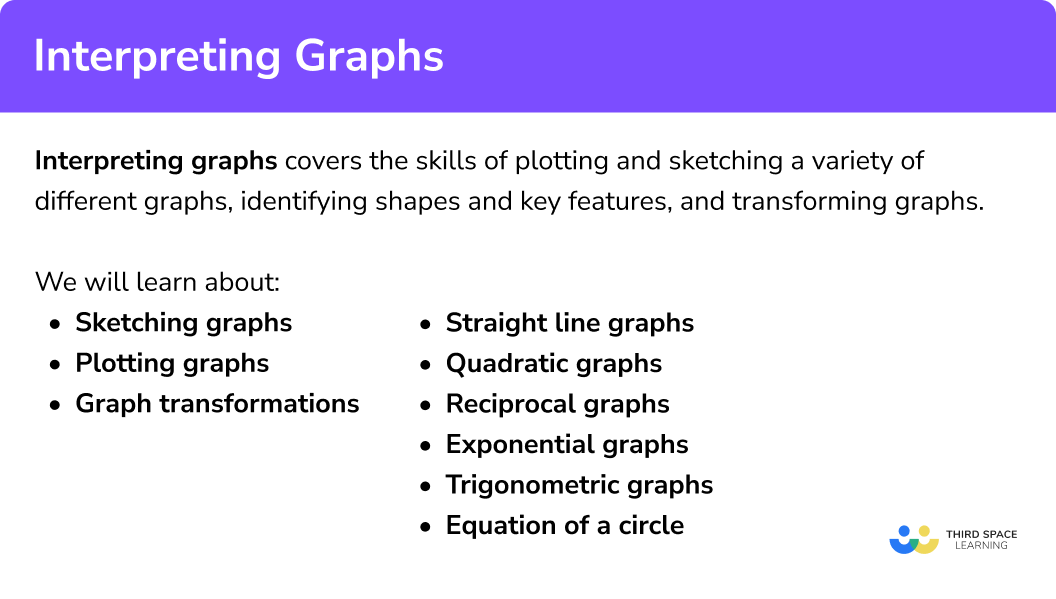### Shapes of different graphs

Different types of graphs have specific shapes and we can recognise the type of graph based on its shape.

For GCSE you need to know the shapes of the following graphs:

Special cases

Graphs of the form x=a are vertical lines and graphs of the form y=a are horizontal lines.

### Key features of graphs

• \textbf{y} intercept

The y intercept of any graph is where it crosses the y axis.

For linear, quadratic and cubic graphs, the y intercept is the constant term in the equation.

For example, the graph of y=x^{2}+3x-4 has a y intercept of -4, meaning it crosses the y axis at -4. This is because when we substitute x=0 into the equation, y=-4, giving us the coordinate (0,-4).

The gradient of a graph is how steep the graph is. It is calculated using the formula

\text{Gradient }=\frac{\text{change in y}}{\text{change in x}}.

A positive gradient slopes upwards from left to right and a negative gradient slopes downwards.

For graphs of linear equations, of the format y=mx+c, the gradient is the value of m.

For curved graphs, we can estimate the gradient of the graph at a certain point by drawing a tangent to the graph at that point and finding the gradient of the tangent.

• Roots ( \textbf{x} intercepts)

The roots, or x intercepts of a graph are the point/points where the graph crosses the x axis. We can find these points by setting y equal to 0 and then solving the equation.

For example, to find the roots of the graph y=x^{2}+5x-14, we set y equal to 0 giving

\begin{aligned} x^{2}+5x-14&=0\\\\ (x+7)(x-2)&=0 \end{aligned}

x=-7 \ or \ x=2

The graph crosses the x axis at -7 and 2.

• Turning points

On a graph, a turning point is a minimum or maximum point on the graph.

For a quadratic graph, the turning point can be found by completing the square.

For example, for the graph y=x^{2}+4x-5, we can complete the square.

\begin{aligned} y&=x^{2}+4x-5\\\\ y&=(x+2)^{2}-4-5\\\\ y&=(x+2)^{2}-9 \end{aligned}

The turning point is the minimum point of this function, which occurs when the bracket is equal to 0. For this function, the bracket is equal to 0 when x=-2 and this gives y=-9. The turning point of this function is therefore (-2, \ -9).

• Asymptote

An asymptote is a line that a graph approaches, without ever touching. The graph gets closer and closer to the line without ever reaching it. We see asymptotes on reciprocal graphs. The blue lines below are asymptotes.

### Plotting graphs

Graphs can be plotted by creating a table of values and plotting the coordinates that are generated.

For example, let’s plot the graph y=2x+1.

The values that we choose to use for x will depend on the type of function that we are plotting. For a linear function, a good range of x values are often -3 to 3. We can then use the formula, in this case y=2x+1, to calculate the y values.

Once we have a table of values, we can plot the pairs of coordinates.

The x value represents the position on the horizontal axis and the y value represents the position on the vertical axis.

Step-by-step guide: Plotting graphs

### Sketching graphs

A sketch of a graph is a rough drawing of the graph, drawn using some of the key features of the graph. For sketches, we don’t usually label the axis, we just mark the coordinates of key points on the graph.

We can sketch graphs using the following information.

• Linear graph: gradient m, and y intercept, c
• Quadratic graphs: y intercept, roots of the graph, coordinates of any turning points
• Cubic graphs: y intercept, roots of the graph
• Reciprocal graph: equations of any asymptotes, the quadrants the graph is in
• Exponential graph: y intercept, value of the base number (greater than or less then 1 )
• Graph of a circle: radius

For example, we can sketch the graph of y=2x^{3}+4x^{2}.

By setting x=0, we can find the y intercept of the graph.

y=2 \times 0^{3} + 4 \times 0^{2}=0

By setting y=0 we can find any x intercepts.

\begin{aligned} 2x^{3}+4x^{2}&=0\\\\ 2x^{2}(x+2)&=0 \end{aligned}

x=0 \ or \ x=-2

We can use this information and the fact that the coefficient of x^3 is positive to sketch the graph.

Step-by-step guide: Sketching graphs

### Transformations of graphs

We can perform different transformations, including translations and reflections, on graphs. Performing a transformation affects the equation of the function in a specific way.

If we have a function, y=f(x), we can apply the following transformations.

Step-by-step guide: Graph transformations

## Interpreting graphs examples

### Example 1: plotting a graph

Plot the graph of y=x^{3}-2x+1.

1. Create a table, fill in suitable values for \textbf{x} and use the equation of the graph to complete the values for \textbf{y} .

2Plot the coordinates on the axes.

3Join the coordinates.

The points should be joined with a smooth curve.

### Example 2: recognising the shape of a graph

Which is the correct equation for this graph?

### Example 3: equation of a linear graph

Find the equation of this straight line.

Find the gradient of the line.

Identify the \bf{y} intercept of the line.

Write the equation of the line.

### Example 4: features of a quadratic graph

Find the coordinates of any points of intersection with the axes and the coordinates of the turning point of the graph y=x^{2}+6x-16. Use these coordinates to sketch the graph.

Find the \textbf{y} intercept by setting \bf{x=0.}

Find the roots of the graph by setting \bf{y=0.}

Find the turning point by completing the square.

Sketch the graph.

### Example 5: sketch a reciprocal

Sketch the graph of y=\frac{3}{x}.

Identify any asymptotes.

Decide which quadrants the graph is in.

Sketch the graph.

### Example 6: sketch the graph of a circle

Sketch the circle x^{2}+y^{2}=16.

Identify the radius of the circle.

Sketch the circle.

### Example 7: transformation of a graph

Here is a sketch of the graph y=\cos(x). On the same diagram, draw the graph of y=\cos(x)+1.

Determine whether the transformation is a translation or reflection.

Choose the correct transformation to apply from the rules:

Write down the required coordinate or sketch the graph.

### Common misconceptions

• Substituting values when plotting graphs

Take care when substituting values, especially negatives.

• Straight line or curve

The points of a linear graph should be joined with a straight line but the points of any other graph should be joined with a smooth curve.

Remember that a positive gradient is one which slopes upwards whereas a negative gradient slopes downwards.

• Translating a graph

The transformation f(x+a) moves the graph left by a units (a translation of -a ).

### Practice interpreting graphs questions

1. Which table shows the correct values for the graph y=x^2+1?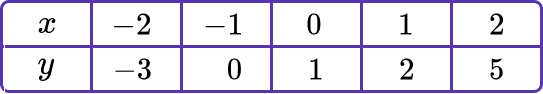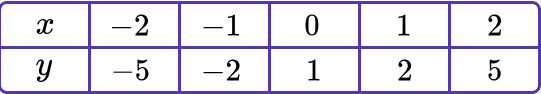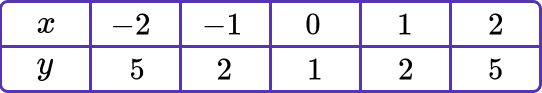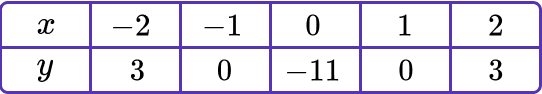(-2)^2+1=4+1=5

(-1)^2+1=1+1=2

(0)^2+1=0+1=1

(1)^2+1=1+1=2

(2)^2+1=4+1=5

2. Which diagram shows the graph of y=\frac{2}{x}?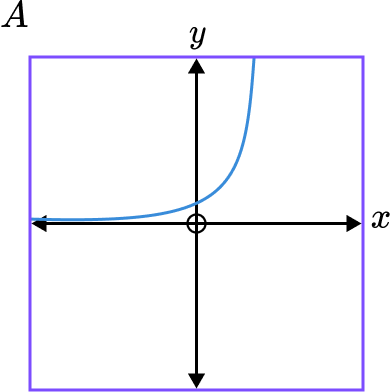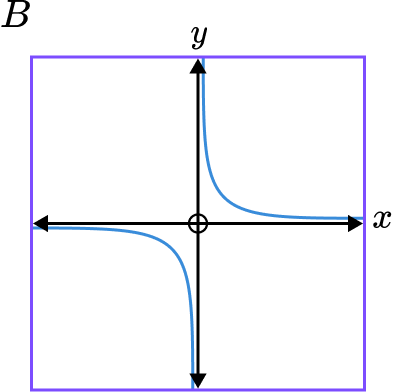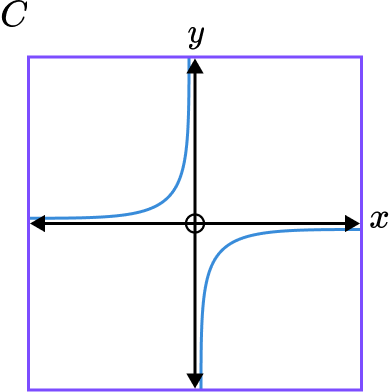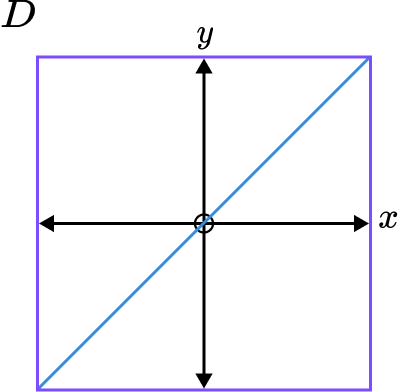Graphs B and C are reciprocal graphs. We are looking for a positive reciprocal graph so it will be in quadrants 1 and 3. This is graph B .

3. What is the equation of this graph?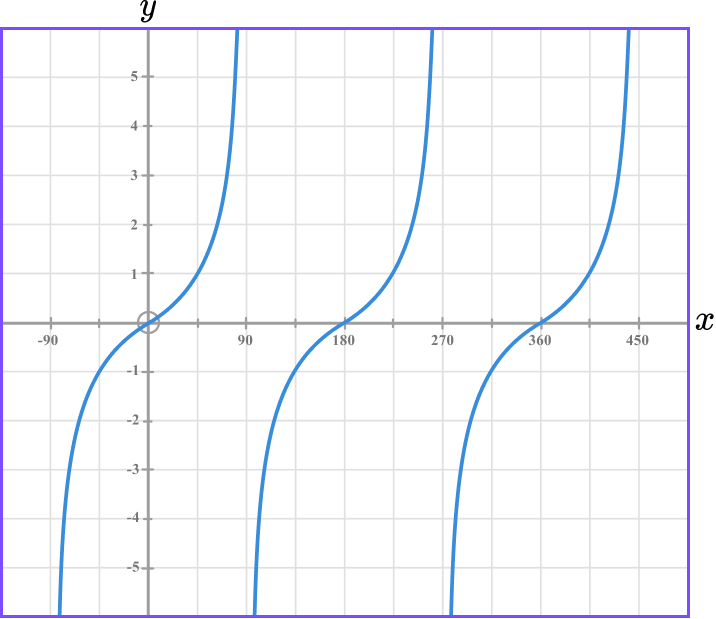y=\sin(x)y=\cos(x)y=\tan(x)x^{2}+y^{2}=90This is a trigonometric graph with asymptotes occuring every 180 degrees from 90^{\circ}. The sine and cosine functions are continuous smooth curves and so this is the graph of y=\tan(x).

4. Which of these is not a point of intersection with the axes for the graph y=x(2x^{2}-8)?

(-2, \ 0)(2, \ 0)(0, \ 0)(8, \ 0)To find the points of intersection, let x=0 and y=0.

When x=0, \ y=0\times(2 \times 0^{2}-8)=0.

So (0, 0) is a point of intersection.

When y=0,

x(2x^{2}-8)=0

x=0

or

\begin{aligned} 2x^{2}-8&=0\\\\ 2x^{2}&=8\\\\ x^{2}&=4\\\\ x&=\pm 2 \end{aligned}

So (2, \ 0) and (-2, \ 0) are points of intersection.

5. Which of these shows a sketch of the graph y=4x-2?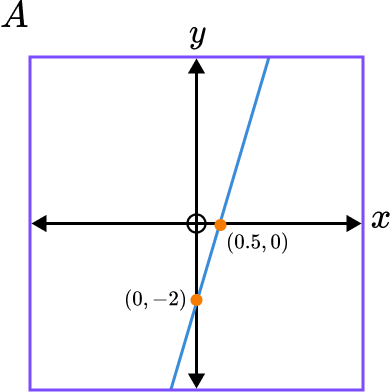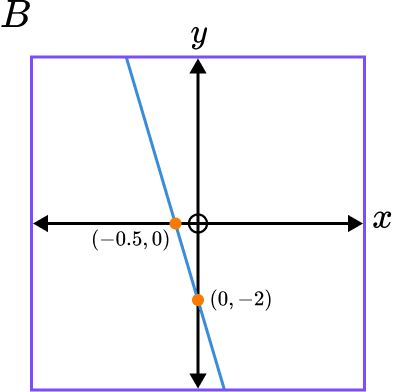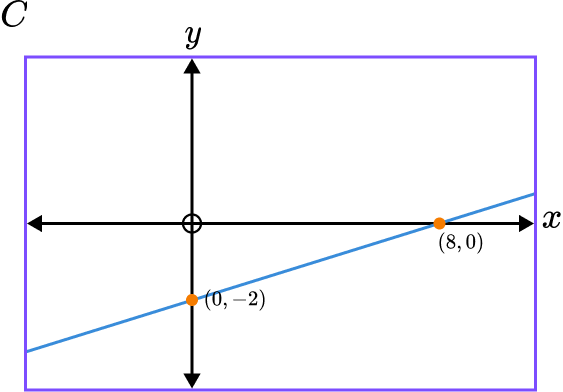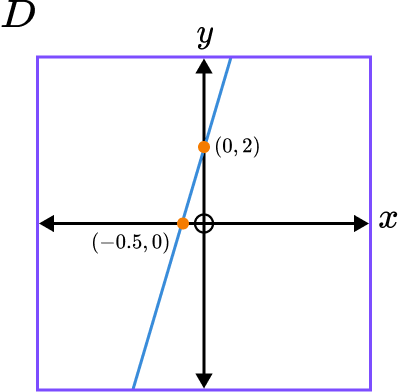The gradient of the line is 4 and the y intercept is -2.

Graph A has a positive gradient of 4 and intercepts the y axis at -2.

6. This is the graph of y=2^{x}. Which transformation is applied to give the graph y=2^{-x}?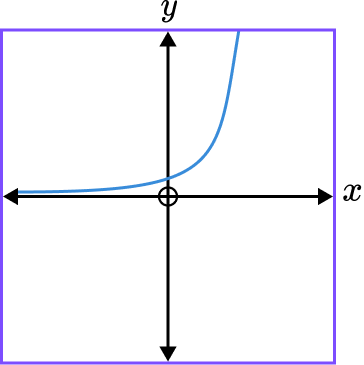Reflection in the x axis.Reflection in the y axis.Translation of -1 in the y direction.Translation of 1 in the x direction.y=2^{-x} is of the form f(-x) so is a reflection in the y axis.

### Interpreting graphs GCSE questions

1. (a) Complete the table of values for y=0.5x+1.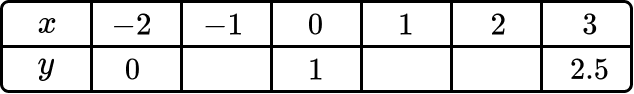(b) Plot the graph y=0.5x+1 on the grid below.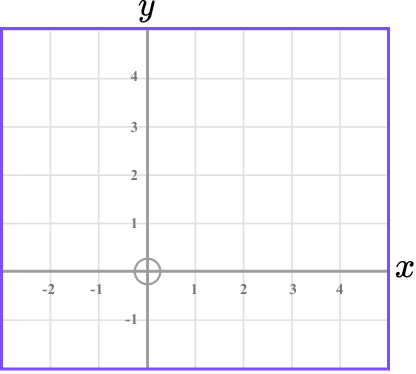(4 marks)

(a)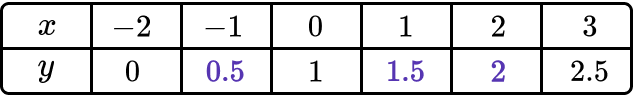At least 2 values are correct.

(1)

All three values are correct.

(1)

(b)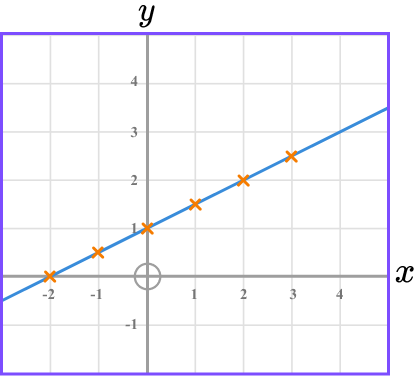At least 4 points plotted correctly.

(1)

All points plotted and joined with a straight line.

(1)

2. Write the correct letter of the equation for each graph.

A: y=2x-3 \hspace{0.4cm} B: y=x^2-3 \hspace{0.4cm} C: y=x^3+2 \hspace{0.4cm} D: y=3-2x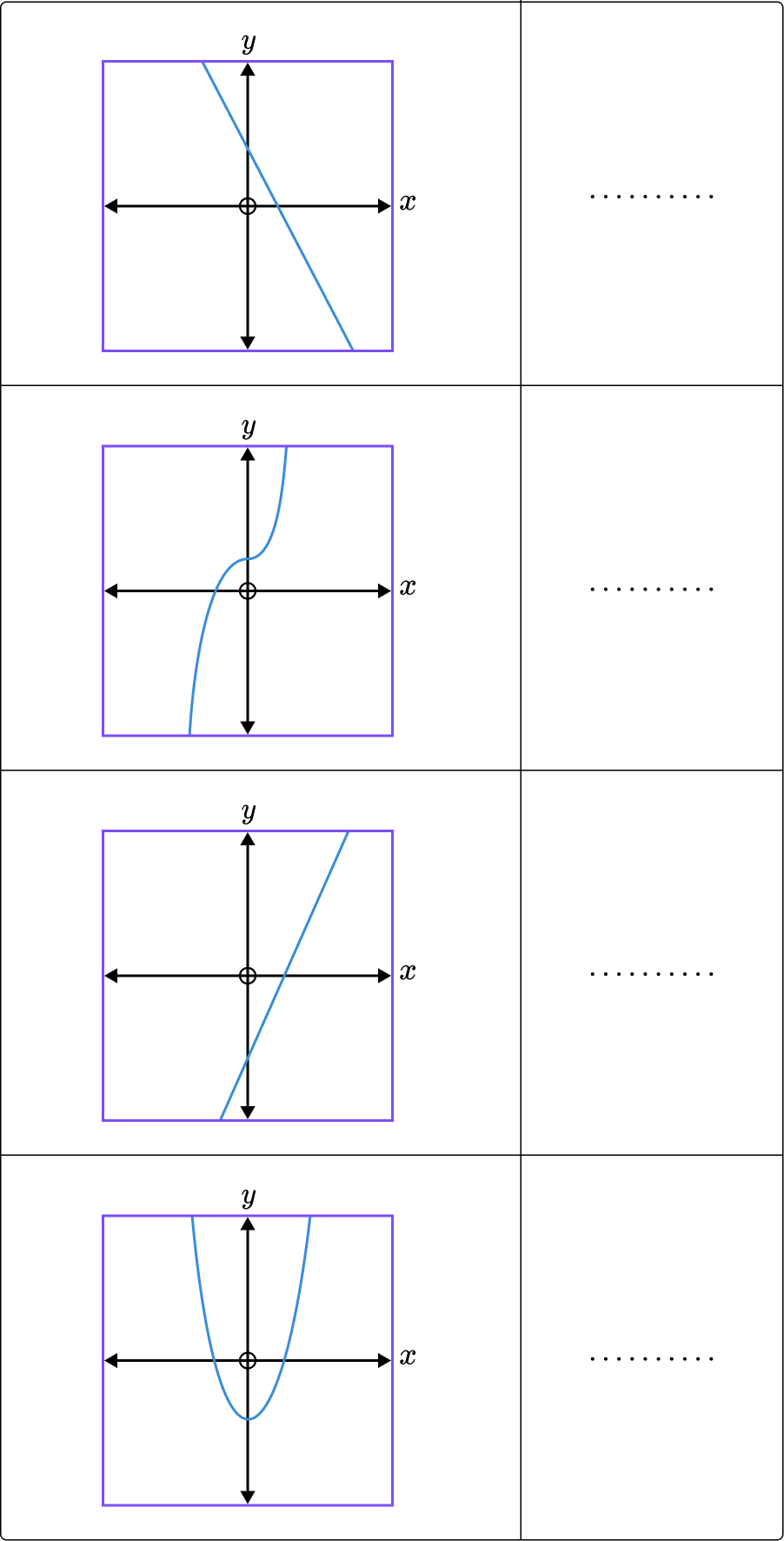(2 marks)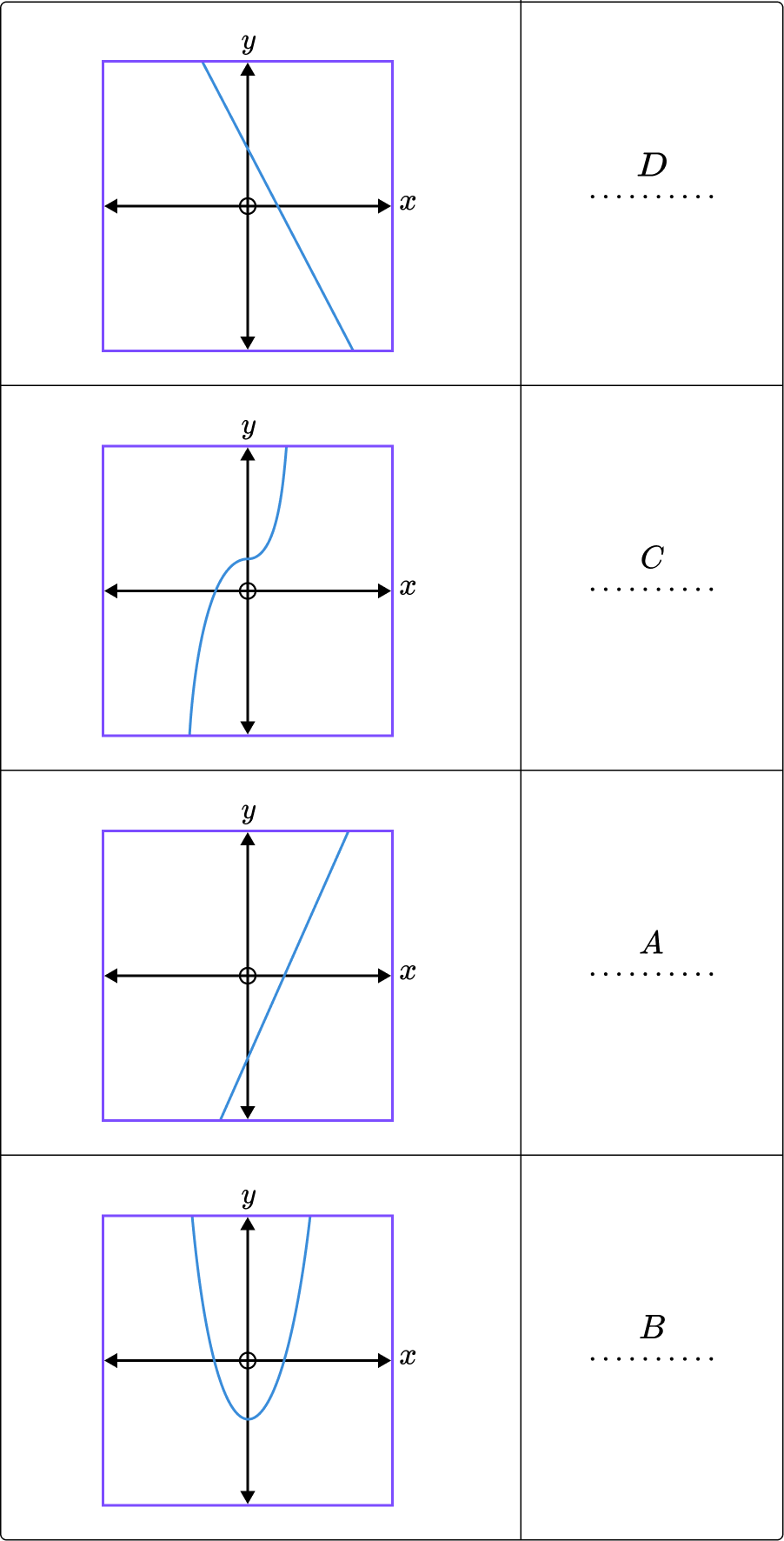At least 2 are correct.

(1)

All 4 are correct.

(1)

3. Sketch the graph of y=x^{2}+4x. Label any points of intersection with the axis and the turning point of the graph.

(4 marks)

y=0^{2}+4\times{0}=0

(1)

x^{2}+4x=0, \ x=0 \ or \ x=-4

(1)

y=(x+2)^{2}-4, minimum point: (-2, \ -4)

(1)

Labelled sketch

(1)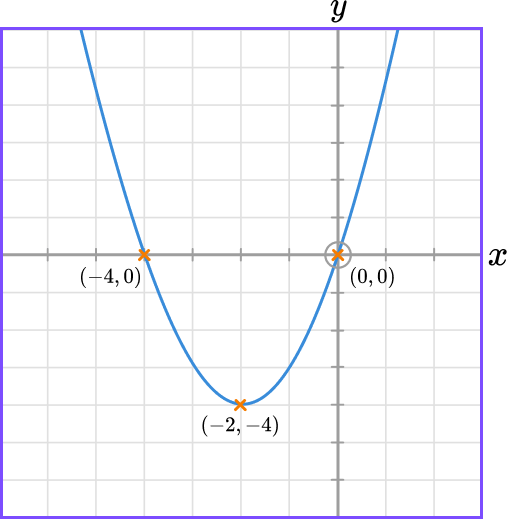## Learning checklist

You have now learned how to:

• Plot a graph
• Interpret line graphs and other graphs
• Recognise different graphs
• Identify key features of a graph
• Sketch a graph
• Transform a graph

## Still stuck?

Prepare your KS4 students for maths GCSEs success with Third Space Learning. Weekly online one to one GCSE maths revision lessons delivered by expert maths tutors.

Find out more about our GCSE maths tuition programme.